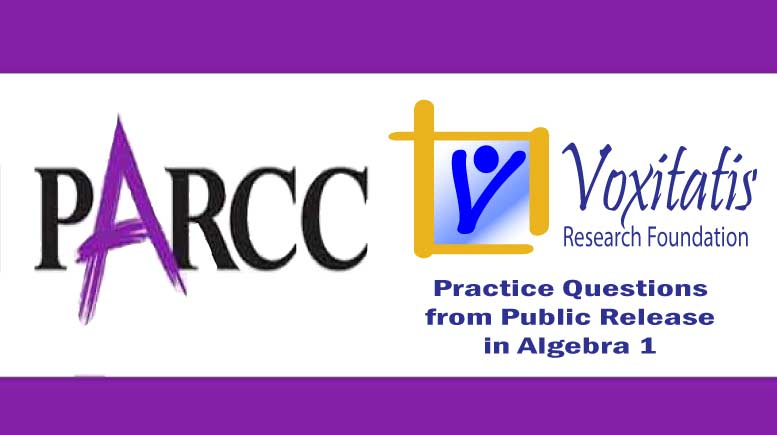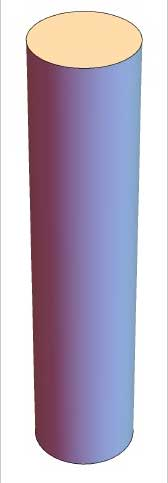Sunday, April 18, 2021# Algebra 1 PARCC question: rewrite formula

-#### The following multiple-choice question, explained here in hopes of helping algebra students in Maryland and Illinois prepare for the PARCC test near the end of this school year, appears on the released version of PARCC’s Spring 2015 test in algebra 1, here:

A set production designer creates a right circular cylindrical pillar. The designer knows the amount of material used for the surface of the pillar and needs to find the height for a reinforcement rod.

Use A = (2πr) h + πr2, where r represents the radius, h represents the height of the pillar, and A represents the surface area of the pillar. What is the formula for h in terms of the other variables that can be used to find the height?

•$h = \frac{A - \pi r^2}{2 \pi r}$
•$h = \frac{A + \pi r^2}{2 \pi r}$
•$h = \frac{A}{3 \pi r^2}$
•$h = \frac{A}{2 \pi r} - \frac{1}{2}$

Answer and references

Correct answer: A,$h = \frac{A - \pi r^2}{2 \pi r}$

PARCC evidence statement(s) tested: A-CED.4-1: Rearrange linear formulas to highlight a quantity of interest, using the same reasoning as in solving equations. For example, rearrange Ohm’s law V = IR to highlight resistance R.

Tasks have a real-world context. The quantity of interest is linear in nature.

The evidence statement above references Math Practice 2, Math Practice 6, and Math Practice 7:

[MP.6] Mathematically proficient students try to communicate precisely to others. They try to use clear definitions in discussion with others and in their own reasoning. They state the meaning of the symbols they choose, including using the equal sign consistently and appropriately. They are careful about specifying units of measure, and labeling axes to clarify the correspondence with quantities in a problem. They calculate accurately and efficiently, express numerical answers with a degree of precision appropriate for the problem context. In the elementary grades, students give carefully formulated explanations to each other. By the time they reach high school they have learned to examine claims and make explicit use of definitions.

The question tests students’ understanding of the Common Core high school algebra standard HSA.CED.A.4 (under Creating Equations that Describe numbers or relationships), which says exactly what the evidence statement above does.

Solution strategy (there are others)

Algebraically rearrange the equation so that h is on one side by itself.

Given this equation for surface area:$A = (2 \pi r)h + \pi r^2$

Subtract πr2 from both sides$A - \pi r^2 = (2 \pi r)h$

Divide by (2πr)$\frac{A - \pi r^2}{2 \pi r} = h$

No numbers are provided, but you can check the reasonableness of this expression by making up some numbers and plugging them in, assuming you know what a right circular cylindrical pillar on a “set” looks like.radius 10″ and height 90″

Let’s say it’s something like the right circular cylindrical pillar shown at the right: This cylinder has a height of 90 inches and a radius of 10 inches. It is not drawn to scale.

Next, compute the surface area using the original formula.$A = (2 \pi (10))(90) + \pi (10)^2$$A \approx (2)(3.14)(10)(90) + (3.14)(10)^2$$= 5652 + 3140 = 8792$

Now put that value into our expression for height and see if we get a height of 90 inches.$h \approx \frac{8792 - (3.14)(10)^2}{(2)(3.14)(10)}$$= \frac{8792 - 3140}{62.8}$$= \frac{5652}{62.8} = 90$

## Resources for further study

Purple Math, developed by Elizabeth Stapel, a math teacher from the St Louis area, has a few pages on solving literal equations for the variable of interest. They begin here. She shows students, say, how to solve the equation for the perimeter of a rectangle, P = 2l + 2w, for w.

Chapter 1, Section 1.4 of the book Algebra 2, Illinois edition by Ron Larson et al is entitled “Rewrite Formulas and Equations” and deals with this type of algebraic manipulation. The authors walk students through solving the equation for the circumference of a circle, given the radius, for the radius, given the circumference.

Complete reference: Ron Larson, Laurie Boswell, Timothy D Kanold, Lee Stiff. Algebra 2, Illinois edition. Evanston, Ill.: McDougal Littell, a division of Houghton Mifflin Company, 2008. The book is used in several algebra classes taught in Illinois high schools.

## Analysis of this question and online accessibility

The question measures knowledge of the content in the Common Core standard it purports to measure and tests students’ ability to rearrange a formula to determine the expression for the variable of interest. It is considered to have a low cognitive demand.

Math Practice 6, which basically says students who are mathematically proficient should attend to precision, such as with the use of an equal sign or units of measurement, is not tested with this question. The question therefore only aligns with the PARCC evidence statement in terms of the content standard, not in terms of the standards for mathematical practice. A possible fix could include adding units of measurement to the problem, although the multiple-choice format hinders that effort to a certain extent.

(The wording of the question is clumsy. For example, the object of the verb creates, pillar, is modified by three adjectives: “right circular cylindrical.” Students who have limited English proficiency may have difficulty with this sentence. A possible fix could include breaking the pillar up: A set designer creates a pillar, which is in the shape of a right circular cylinder. However, the solution process doesn’t depend on an understanding of the pillar, so any misunderstanding of the context is probably moot with respect to testing the standard.)

The question can be tested online and should yield results that are as valid and reliable as those obtained on paper. The multiple-choice format may promote guessing, which casts doubt on the validity of the question. In terms of both Math Practice 6 and guessing, the question would have been better asked in a constructed-response format.

No special accommodation challenges can be identified with this question, so the question is considered fair, except in an auxiliary way for students who have limited proficiency in English. This secondary unfairness may be moot and is due mainly to context issues, such as the use of the term “reinforcement rod,” with which algebra students may have limited experience. It can’t be considered securely held knowledge. Remember, this is an algebra test, not a construction class test. Terminology should be restricted to the domain of algebra.

## Challenge

The formula for the area of a triangle is A = ½bh, where b is the length of the base of the triangle and h is its height. Write the formula for determining the base of a triangle given the area and height. Then, what is the base of a triangle with area 9 cm2 and height 3 cm?

## Purpose of this series of posts

Voxitatis is developing blog posts that address every algebra 1 question released to the public by the Partnership for Assessment of Readiness for College and Careers, or PARCC, in order to help students prepare to take the test this spring.

Our total release will run from February 27 through March 15, with one or two questions discussed per day. Then we’ll move to geometry at the end of March, algebra 2 during the first half of April, and eighth grade during the last half of April.Paul Katulahttps://news.schoolsdo.org
Paul Katula is the executive editor of the Voxitatis Research Foundation, which publishes this blog. For more information, see the About page.

### Md. to administer tests in math, English

0
Students in Md. will still have to take standardized tests this spring in math and English language arts, following action of the state board.